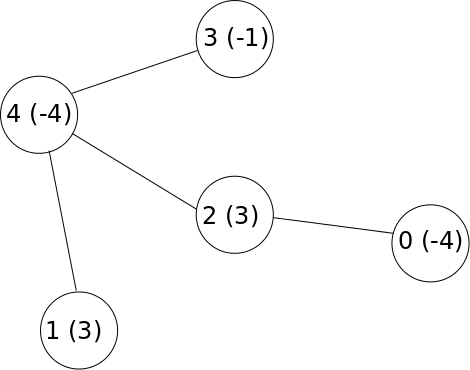시간 제한 메모리 제한 제출 정답 맞은 사람 정답 비율
2 초 1024 MB 0 0 0 0.000%

## 문제

In a far away kingdom, there are $N$ cities numbered between $0$ and $N - 1$. The cities are connected by $N - 1$ two-way roads. Each road connects exactly two cities, such that there is a unique path between any pair of cities. Each city $i$ has some value $C[i]$ (possibly negative).

Unfortunately, the old king of the kingdom died, without appointing a successor. Thus a civil war broke out in the kingdom, between the $P$ lords (also numbered between $0$ and $P - 1$) who wish to gain control of the kongdom.

After a long and terrible war, the lords realized none of them could beat all the other lords in the war. They agreed to a truce, and decided to divide the kingdom into $P$ parts, one part per lord. The parts must be so that if two cities $a$ and $b$ lie in the same part, all cities in the unique path between them must also lie in that part. Since no lord wants to be left out, each one must be given at least one city.

Since no lord wants to get less than another, the value of each part must be the same. The value of a part is the sum of the values of all cities in that part.

## 예제

Let the kingdom have $N = 5$ cities, connected by roads as in the figure below:Figure 1: Illustration of the example

Each city has its value given within parenthesis after the city number.

In total, we wish to divide the kingdom into $P = 3$ parts. Then, a possible subdivision would be $(0, 2)$, $(3)$ and $(1, 4)$. The values of each part would then be $-4 + 3 = -1$, $-1$ and $3 - 4 = -1$, so all parts in this subdivision would have the same value.

Note that $(0, 1)$, $(3)$, $(2, 4)$ would not be an acceptable division even though all parts have the same value. This is because the path between cities $0$ and $1$ is $1, 4, 2, 0$, but cities $4$ and $2$ belong to another part.

## 구현

Your task is to compute a subdivision of the kingdom as described in the statement. You will implement the function division(N, P, C, F, T).

• division(N, P, C, F, T) - this function will be called exactly once by the judge.
• N: the number of cities in the kingdom.
• P: the number of parts we wish to divide the kingdom into.
• C: an array with $N$ elements. C[i] ($0 \le i < N$) contains the value of city $i$.
• F: an array with $N - 1$ elements. F[i] ($0 \le i < N - 1$) contains one of the cities that the $i$:th road connects.
• T: an array with $N - 1$ elements. T[i] ($0 \le i < N - 1$) contains the other city that the $i$:th road connects.
• It is always possible to travel between any pair of cities using the roads.
• $|C[i]| < 10^9$
• The function should return 1 if it is possible to find such a subdivision, and 0 if it is impossible.

Additionally, you should make a single call to function parts(S) to construct your subdivision.

• parts(S) - this function should be called a single time.
• S: an array with $N$ elements. $S[i]$ $(0 \le i < N)$ should contain the number of the lord who will get city $i$.
• The function has no return value.

A code skeleton containing the function to be implemented, together with a sample grader, can be found at attachments.zip.

## 입력

The sample judge reads input in the following format:

• line $1$: N P
• line $2$: C C .. C[N - 1]
• line $3$: F F .. F[N - 2]
• line $4$: T T .. T[N - 2]

## 출력

The sample judge will first write a single line with the return value of division(N, P, C, F, T). The next line will contain $N$ integers containing the input to the parts(R) call.

## 제한

• $1 \le P \le N \le 100\,000$

## 예제 입력 1

5 3
-4 3 3 -1 -4
0 1 2 3
2 4 4 4


## 예제 출력 1

1
0 1 0 2 1


## 출처• 문제를 만든 사람: Johan Sannemo

## 제출할 수 있는 언어

C++17, Java 8, C++14, Java 8 (OpenJDK), Java 11, C++20, Java 15, C++14 (Clang), C++17 (Clang), C++20 (Clang)

## 채점 및 기타 정보

• 예제는 채점하지 않는다.